# Verbal Reasoning - Character Puzzles

### Exercise :: Character Puzzles - Character Puzzles 1

41.

Which one will replace the question mark ?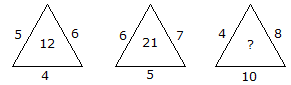A. 14 B. 22 C. 32 D. 320

Explanation:

(5 x 6 x 4)/10 = 12

and (6 x 7 x 5)/10 = 21

Therefore (4 x 8 x 10)/10 = 32.

42.

Which one will replace the question mark ?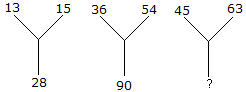A. 18 B. 90 C. 108 D. 28

Explanation:

13 + 15 = 28

36 + 54 = 90

Therefore, 45 + 63 = 108.

43.

Which one will replace the question mark ?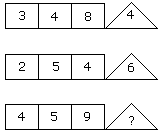A. 8 B. 9 C. 10 D. 11

Explanation:

(3 x 4 - 8) = 4

(2 x 5 - 4) = 6

(4 x 5 - 9) = 11.

44.

Which one will replace the question mark ?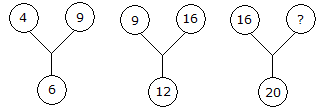A. 60 B. 50 C. 25 D. 21

Explanation: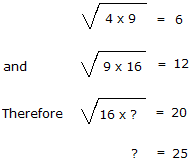45.

Which one will replace the question mark ?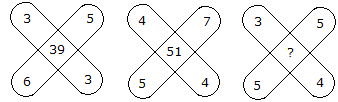A. 47 B. 45 C. 37 D. 35

Explanation:

(3 x 3) + (5 x 6) = 39

and (4 x 4) + (5 x 7) = 51

Therefore, (3 x 4) + (5 x 5) = 37.